# 2nd PUC Chemistry Question Bank Chapter 8 The d-and f-Block Elements

## Karnataka 2nd PUC Chemistry Question Bank Chapter 8 The d-and f-Block Elements

### 2nd PUC Chemistry The d-and f-Block Elements NCERT Textbook Questions and Answers

Question 1.
Write down the electronic configuration of:

1. Cr3+
2. Pm3+
3. Cu+
4. Ce4+
5. CO2+
6. Lu2+
7. Mn2+
8. Th4+

1. Cr3+ : 1 s2 2s2 2p6 3s2 3p6 3d3 4s0 or [Ar] 3d3 4s0
2. Pm3+ : 1s2 2s2 2p6 3s2 3p6 3d10 4s2 4p6 4d10 5s6 5p6 6s0 5d0 4f4
3. Cu+ : 1s2 2s2 2p6 3s2 3p6 3d10 4s0 or [Ar] 3d10 4s0
4. Cr3+ : 1s2 2s2 2p6 3s2 3p6 3d3 4s0 or [Ar] 3d34s0
5. Ce4+ : 1s2 2s2 2p6 3s2 3p6 4s2 3d10 4p6 5s2 4d10 5p6 6s0 5d0 4f0 or [Xe] 4f4 5d0 6s0
6. Co2+ : [Ar] 3d7 4s0
7. Lu2+ : [Xe] 4f14 5d1 6s0
8. Mn2+ : [Ar] 3d5 4s0
9. Th4+ : [Rn] 5f0 6d2 7s2

Question 2.
Why are Mn2+ compounds more stable than Fe2+ towards oxidation to their +3 state?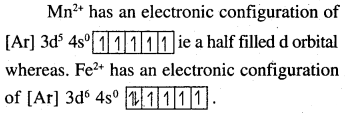Electronic configuration of Mn2+ is 3d5. This is a half-filled configuration and hence stable. Therefore, the third ionization enthalpy is very high, i. e., the third electron cannot be lost easily. Electronic configuration of Fe2+ is 3d6. It can lose one electron easily to achieve a stable configuration 3d5.Question 3
Explain briefly how the +2 state becomes more and more stable in the first half of the first-row transition elements with increasing atomic number?
Except for Sc, all other metals display +2 oxidation state. Also, on moving from Sc to Mn,’ the atomic number increases from 21 to 25; This means the number of e in 3d orbital increases from 1 to 5
Sc2+ : d1 Ti2+ = d2 V2+ = d3 Cr2+ = d4
Mn2+ : d5
+ 2 oxidation state is attained by the loss of 2, 4s electrons by these metals since the number of d electrons in (+2) state also increases from Ti (+2) to Mn (+2), the stability of +2 state increases (as d – orbital is becoming more is half-filled) M+2 has d5 electrons (that is half-filled d shell, which is highly stable).

Question 4.
To what extent do the electronic configurations decide the stability of oxidation states in the first series of the transition elements? Illustrate your answer with examples.
The presence of half-filled or completely filled orbitals imparts stability to a particular element/ion. The greater the number of such orbitals the more will be the relative stability. For example, let us write the different oxidation states of Mn (Z = 25) along with the electronic configurations. Mn: [Ar] 3d54s2 ; Mn2+ ; [Ar] 3d5 , Mn3+ ; [Ar]3d4, Mn4+ ; [Ar] 3d3.

+2 oxidation state of the element is likely to be the most stable because the corresponding electronic configuration of Mn2+ is highly symmetrical (all the five 3d-orbitals are half-filled).

Question 5.
What may be the stable oxidation state of the transition element with the following d electron configurations in the ground state of their atoms: 3d3, 3d5,3d8, and 3d4?Question 6.
Name the oxometal anions of the first series of the transition metals in which the metal exhibits the oxidation state equal to its group number.
Upto the element Mn, the oxidation state leading to the maximum stability corresponds to a value equal to the sum of s- and d-electrons i.e., the group number. For example,
[Sc(III)O2], [Ti(IV)O3]2-, [V(V)O3], [Cr(VI)O4]2-, [Mn(VII)O4]
However, for the remaining elements, the oxidation states are not related to their group numbers.

Question 7.
What is lanthanoid contraction? What are – the consequences of lanthanoid contraction?
The regular decrease in atomic radii due to the filling of 4f before 5d orbitals is called lanthanoid contraction. This is because of the imperfect shielding of one electron by another in the same set of orbitals. The shielding of one 4f electron by another is less than that of one d electron by another, and as the nuclear charge increases along the series, there is fairly regular decrease in the size of entire 4f orbitals. Consequences of lanthanoid contraction.

• Radii of third series are virtually same as those of the corresponding member of second series.
• Third series and second series have very similar physical and chemical properties much more than that expected by usual family relationships.

Question 8.
What are the characteristics of the transition elements and why are they called transition elements? Which of the d-block elements may not be regarded as the transition elements?
General characteristics of transition elements.

1. Electronic configuration – (n – 1) d1-10 ns1-2
2. Metallic character – With the exceptions of Zn, Cd, and Hg, they have typical metallic structures.
3. Atomic and ionic size – Ions of the same charge in a given series show a progressive decrease in radius with increasing atomic number.
4. Oxidation state – Variable ; ranging from + 2 to + 7.
5. Paramagnetism – The ions with unpaired electrons are paramagnetic.
6. Ionisation enthalpy – Increases due to increase in molecular charge.
7. Formation of coloured ions – Due to unpaired electrons.
8. Formation of complex compounds – Due to small size and high charge density of metal ions.
9. They possess catalytic properties – Due to their ability to adopt multiple oxidation states.
10. Formation of interstitial compounds.
11. Alloy formation.

They are called transition elements due to their incompletely filled d-orbitals in the ground state or any stable oxidation state and they are placed between s and p-block elements. Zn, Cd, and Hg have fully filled d° configuration in their ground state hence may not be regarded as the transition elements.Question 9.
In what way is the electronic configuration of the transition elements different from that of the non-transition elements?
The general electronic configuration of a d-block elements is (n – 1) d1-10 ns1-2.
Transition elements in their ground state or excited state will have a configuration of (n – 1) d1-9 ns0-2 ie. partly filled d orbitals whereas non-transition elements will have a configuration of (n – 1) d10 ns0.2  i.e completely filled d- orbitals.

Question 10.
What are the different oxidation states exhibited by the lanthanoids?
The common stable oxidation state of lanthanoids is + 3. However, some may also exhibit + 2 and + 4 oxidation states.

Question 11.
Explain giving reasons:
(i) Transition metals and many of their compounds show paramagnetic behaviour.
(ii) The enthalpies of atomisation of the transition metals are high.
(iii) The transition metals generally form coloured compounds. .
(iv) Transition metals and their many compounds act as good catalyst.
(a) Paramagnetism: arises from the presence of unpaired electrons, each such electron has magnetic moment associated with its spin angular momentum and orbital angular momentum. For the compounds of the first series of transition metals, the contribution of the orbital angular momentum is effectively quenched and hence is of no significance. For these, the magnetic moment,is determined by the number of unpaired electrons and is calculated by using the ‘spin’ only’ formula, i.e., µ = $$\sqrt { n(n+2) }$$ B.M

(b) Enthalpy of atomisation: may be defined as the amount of heat energy needed to break the metal lattice of a crystalline metal into free atoms. Greater the magnitude of lattice energy more will be the value of enthalpy of atomisation. The transition metals have high enthalpies of atomisation because the metallic bonds present are quite strong due to the presence of large number of half-filled atomic orbitals. For more details, consult text-part.

(c) Due to the presence of unpaired electrons and d-d transitions: the transition metals are generally coloured. When an electron from a lower energy d orbital is excited to a higher energy d-orbital, the energy of excitation corresponds to the frequency of light absorbed. This frequency generally lies in the visible region. The colour observed corresponds to the complementary colour of the light absorbed. The frequency of the light absorbed is determined by the nature of the ligand.

(d) The transition metals and their compounds are known for their catalytic activity: This activity is ascribed to their ability to adopt multiple oxidation states and to form complexes. Vanadium(V) oxide (in Contact Process), finely divided iron (in Haber’s Process), and nickel (in r Catalytic Hydrogenation) are some of the examples. Catalysts at a solid surface involve the formation of bonds between reactant molecules and atoms of the surface of the catalyst.

Question 12.
What are interstitial compounds? Why are such compounds well known for transition metals?
Interstitial compounds are those in which small atoms occupy the interstitial sites in the crystal lattice. Transition metals can accommodate small atoms like H, B, C, N, etc. in their voids in the crystal lattices and hence form interstitial compounds.

Question 13.
How is the variability in oxidation states of transition metals different from that of non-transition metals? Illustrate with examples.
The variability of oxidation states, a characteristic of transition elements, arises due to incomplete filling of d-orbitals in such a way that their oxidation states differ from each other by unity, e.g., Fe2+, Fe3+, Cr2+, Cr3+. This is in contrast with the variability of oxidation states of non-transition elements where oxidation states normally differ by a unit of two. i.e., Sn2+, Sn4+, P3+ and P5+, etc.
in the p-block the lower oxidation states are favoured by the heavier members (due to inert pair effect), the opposite is true in the groups of J-block.For example, in group 6, Mo (VI) and W (VI) are found to be more stable than Cr (VI). Thus Cr (VI) in the form of dichromate in acidic medium is a strong oxidising agent, whereas MOO3 and WO3 are not.

Question 14.
Describe the preparation of potassium dichromate from iron chromite ore. What is the effect of increasing pH on a solution of potassium dichromate?
Potassium Dichromates are generally prepared from chromates which in turn are obtained by the fusion of chromite ore (FeCr2O4) with sodium or potassium carbonate in free excess of air

4FeCr2O4 + 8Na2CO3 + 7O2 → 8Na2CrO4 + 2Fe2O3 + 8CO2

The yellow solution of Na2CrO4 is filtered and acidified with H2SO4 to give a solution from which orange sodium dichromate Na2Cr2O7 2H2O can be crystallised
2Na2CrO4 + 2H+→ Na2Cr2O7 + 2Na+ + H2O

sodium dichromate is more soluble than potassium dichromate. The latter is prepared by treating the solution of Na2Cr2O4 with KCl.

Na2Cr2O7 + 2KCl → K2Cr2O7 + 2NaCl (orange crystals)

(b) On increasing the PH of a solution of K2Cr2O7, it behaves like a strong oxidising agent and it oxidises iodide to iodine sulphides to sulphur, tin (II) to tin (IV) and Iron (II) salts to iron (III).

Question 15.
Describe the oxidising action of potassium dichromate and write the ionic equations for its reaction with:
(i) iodide (ii) iron(II) solution and (iii) H2S
Potassium dichromate, in acidic medium acts as a strong oxidising agent by gaining 6e’ and forms Cr3+.
Cr2O72- + 14H+ + 6e → 2Cr3+ + 7H2O ..(1)
(i) iodides
6I → 3I2 + 6e ..(2)
(1) + (2)
Cr2O72- + 14 H+ + 6I → 2Cr3+ + 3I2 + 7H2O
(ii) iron (II) solution
6Fe2+ → 6Fe3+ + 6e ..(3)
(1) + (3)
Cr2O72- +14 H++ 6Fe2+ → 2Cr3+ + 6Fe3+ + 7H2O

(iii) H2S
3H2S → 6H+ + 3S + 6e ..(4)
(1) + (4)
Cr2O72- +14H+ + 3H2S → 6H+ + 3S + 2Cr3+ + 7H2OQuestion 16.
Describe the preparation of potassium permanganate. How does the acidified permanganate solution react with
(i) iron(II) ions
(ii) SO2 and
(iii) oxalic acid?
Write the ionic equations for the reactions.
Potassium permangnate is prepared by fusion of MnO2 with an alkali metal hydroxide and an oxidising agent like KNO3. This produces the dark green k2MnO4 which disproportionates in a neutral or acidic solution to give permanganate.

2MnO2 + 4 KOH + O2 → 2K2MnO4 + 2H2O
3MnO42- + 4H+ → 2MnO4 + MnO2 + 2H2O

Commercially it is prepared by the alkaline oxidative fusion of MnO2 followed by the electrolytic oxidation of manganate (VI)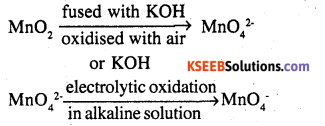In laboralory manganese (II) ion salt is oxidised by peroxidisulphate to permanganate.

2Mn2+ + 5S2O82- + 8H2O → 2MnO4 + 10SO42- + 16H+
(i) 5Fe2+ + MnO4 + 8H+ → Mn2+ + 4H2O + 5Fe37

(ii) 2MnO42+ 10SO2 + 5O2 + 4H2O → 2Mn2+ + 10SO42- + 8H+

(iii) 5C2O42- + 2MnO4 + 16H+ → 2Mn2+ + 8H2O + 10CO2

Question 17.
For M2+/M and M3+/M2+ systems the Eθ values for some metals are as follows:
Cr2+/Cr -0.9V
Mn2+/Mn -1.2V
Fe2+/Fe -0.4V
Cr3+/ Cr2+ -0.4 V
Mn3+/Mn2+ + 1.5 V
Fe3+/Fe2+ + 0.8 V
Use this data to comment upon:
(i) the stability of Fe3+ in acid solution as compared to that of Cr3+ or Mn3+ and
(ii) the ease with which iron can be oxidised as compared to a similar process for either chromium or manganese metal.
(i) From the above data, we can infer that Fe3+ is less stable than Cr3+ but more stable than Mn3+. If reducing potential is less than oxidised state will be more stable. This is so because it is easier to add an e to Fe3+ than Cr3+ but difficult to do so in case of Fe3+ than inMn3+.
(ii) Mn and Cr can be oxidised easily as compared to Fe because the reducing potential of Fe is less negative compared to Mn and Cr. Hence Fe is stable in its reduced form.

Question 18.
Predict which of the following will be coloured in aqueous solution?
Ti3+, V3+, Cu+, Sc3+, Mn2-, Fe3+ and CO2+.
Give reasons for each.
Among the above-mentioned ions, Ti3+, V3+, Mn2+, Fe3+ and Co2+ are coloured. These ions are coloured due to the presence of unpaired electrons, they can undergo d-d transitionsQuestion 19.
Compare the stability of +2 oxidation state for the elements of the first transition series.
The stability of the +2 oxidation state decreases from left to right except for Mn and Zn. Stability decreases towards the right due to decreasing negative value of standard reduction potential. The decrease in the -ve value of E° is due to an increase in the sum of ∆i H1 + ∆iH2 (first and second ionisation enthalpy)

Question 20.
Compare the chemistry of lanthanoids and actinoids with special reference to :
(a) electronic configuration
(b) oxidation state
(c) atomic and ionic sizes
(d) chemical reactivity.
(a) Electronic configuration: The actinoids involve the gradual filling of 5f-subshell in their atoms. Actinium has the outer electronic configuration of 6d17s2. From thorium (Z = 90) onward 5f-subshell gets progressively filled. Because of almost equal energies of 5fand 6d-subshells, there are some doubts regarding the filling of 5fand 6d-subshells.

(b) Oxidation states Members of the actinoid family exhibit more variable oxidation states as compared to the elements belonging to lanthanoid family. This is due to the reason that there is less energy difference in 5d, 6fand 7s orbitals belonging to the actinoid family than the energy difference in 5f, 4d and 65 orbitals in case of lanthanoid family.

(c) Atomic and ionic sizes: The atomic size of lanthanoids decreases from lanthanum to lutetium. Though the decrease is not regular, in case of atomic radii, the decrease in the ionic size (M3+) is regular. Decrease in size between two successive elements is higher in actinoids due to poor screening by 5f electrons.

(d) Chemical reactivity: Actinoids are highly reactive metals especially when these are in finely divided form. Upon boiling with water, they form respective oxides and hydroxides. They combine with a variety of non-metals at moderate temperature. On reacting with hydrochloric acid, they form their respective chlorides.

Question 21.
How would you account for the following:
(i) Of the d4 species, Cr2+ is strongly reducing while manganese (III) is strongly oxidising.
(ii) Cobalt (II) is stable in aqueous solution but in the presence of complexing reagents, it is easily oxidised.
(iii) The d1 configuration is very unstable in ions.
(i) Cr+2 is reducing in nature as its configuration changes from d4 to d3 (A stable configuration having half-filled t28 orbitals). On the other hand, Mn+3 is oxidising in nature as the configuration changes from d4 to d5 (A stable configuration having half-filled t2g to eg orbitals)
(ii) Strong ligands force Cobalt (II) to lose one more electron from 3cfsubshell and thereby induce d2sp3-hybridization.
(iii) The ions with the d1 configuration try to lose the only electron on the d-subshell in order to acquire a stable inert gas configuration.Question 22.
What is meant by ‘disproportionation’? Give two examples of disproportionation reaction in an aqueous solution.
It is found that sometimes a relatively less stable oxidation state undergoes an oxidation-reduction reaction in which it is simultaneously oxidised and reduced. This is called disproportion.
For example,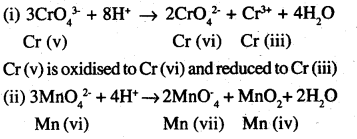Mn (vi) is oxidised to Mn (vii) and reduced to Mnfiv).

Question 23.
Which metal in the first series of transition metals exhibits +1 oxidation state most frequently and why?
Cu has electronic configuration 3 d10 4s1. It can easily lose 4s1 electrons to acquire the stable 3d10 configuration. Hence, it shows + 1 oxidation state.

Question 24.
Calculate the number of unpaired electrons in the following gaseous ions: Mn3+, Cr3+, V3+, and Ti3+. Which one of these is the most stable in an aqueous solution?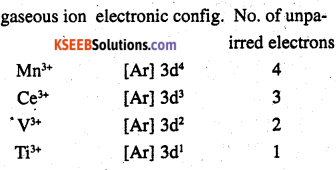Cr3+ is the most stable in an aqueous solution owing to a t23 g configuration.

Question 25.
Give example and suggest reasons for the following features of transition metal chemistry.
(a) The lowest oxide of the transition metal is basic while the highest is acidic.
(b) A transition metal exhibits higher oxidation states in oxides and fluorides. (C.B.S.E. Sample Question Paper 2012)
(c) The highest oxidation state is exhibited in oxoanions of a metal.
(a) It may be noted that in the different oxides of the same element, the acidic strength increases with the increase in oxidation state of the element. MnO (Mn2+) is basic while Mn2O7 (Mn7+) is acidic in nature.
(b) Both oxygen and fluorine being highly electronegative can increase the oxidation state of a particular transition metal. In certain oxides, the element oxygen is involved in multiple bonding with the metal and this is responsible for the higher oxidation state of the metal. For detail, consult text part.
(c) This is also because of the high electronegativity of oxygen. For example, chromium exhibits oxidation state of (VI) in oxoanion [Cr(VI)O4]2- and manganese shows oxidation state of (VII) in oxoanion [Mn(VII)O4].Question 26.
Indicate the steps in the preparation of:
(i) K2Cr2O7 from chromite ore.
(ii) KMnO4 from pyrolusite ore.
(i) Potassium dichromate : (K2Cr2O7) is prepared from chromite ore (FeCl2O4) in the following steps.

step (1) Preparation of sodium chromate
4Fe Cr2O4 + 16 NaOH + 7O2 → 8Na2CrO4 + 2Fe2O3 + 8H2O

step (2) Conversion of sodium chromate into sodium dichromate
2Na2CrO4 + con H2SO4 → Na2Cr2O7 + Na2SO4 + H2O

step (3) Conversion of sodium dichromate to potassium dichromate
Na2Cr2O7 + 2KCl → 2Nacl + k2Cr2O7

potassium chloride is less soluble than sodium chloride is obtained in the form of orange coloured crystals and can be removed by filtration.

The dichromate ion (Cr2O72- ) exists in equilibrium with chromate (CrO42- ) ion at pH.
4. However, by changing the pH, they can be interconverted.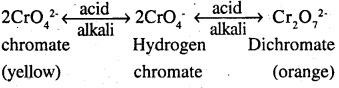(ii) Potassium permanganate : (KMnO4) can be prepared from pyrolusite (MnO2). The ore is fused with KOH in the presence of either atmospheric oxygen or an oxidising agent, such as KNO3 or KClO4 to give K2MnO4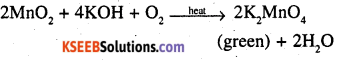The green mass can be extracted with water and then oxidised either electrolytically or bypassing chlorine/ozone into the solution, electrolytic oxidation
K4MnO4 2K++ ↔️ MnO42-
H2O ↔️ H+ + OH
at anode manganate ions are oxidized to permanganate ionsoxidation by chlorine
2K2MnO4 + Cl2 →  2KMnO4 + 2KCl
2MnO42- + Cl2 → 2 MnO4 + 2Cl

oxidation by ozone
2K2MnO4 + O3 + H2O → 2KMnO4 + 2KOH + O2
2MnO42- + O3 + H2O → 2MnO2 + 2OH + O2Question 27.
What are alloys? Name an important alloy which contains some of the lanthanoid metals. Mention its uses.
An alloy is a homogeneous mixture of two or more metals and non-metals. An important alloy containing lanthanoid metals is mischmetal which contains 95% lanthanoid metals (Ce, La, and Nd) and 5% iron along with traces of S, C, Ca and Al. It is used in making parts of jet engines.

Question 28.
What are inner transition elements? Decide which of the following atomic numbers are the atomic numbers of the inner transition elements: 29, 59, 74, 95, 102, 104.
Inner transition metals are those elements in which the last electron enters the f-orbital. The elements in which the 4f and the 5f orbitals are progressively filled are called f-block elements. Among the given atomic numbers the atomic numbers of the inner transition u elements are 59, 95, and 102.

Question 29.
The chemistry of the actinoid elements is not so smooth as that of the lanthanoids. Justify this statement by giving some examples of the oxidation state of these elements.
The lanthanoids mostly exhibit +3 oxidation states in their compounds. Actinoid elements also normally show +3 oxidation states. But in their cases, 5f, 6d, and 7s energy levels are comparable and have very small energy differences in them. As a result, they can exhibit a number of oxidation states. For example,
Neptunium (Np) : +3, +4, +5, +6, +7
Plutonium (Pu) : +3, +4, +5, +6, +7
Americium (Am) : +3, +4, +5, +6.

Question 30
Which is the last element in the series of actinoids? Write the electronic configuration of this element. Comment on the possible oxidation state of this element.
The last element in the actinoid series is Lawrencium. Lr. Its atomic number is 103 and its electronic configuration is [Rn] 5f14 6d17s2. The most common oxidation state displayed by it is +3 because after losing 3 electrons it attains a stable f14 configuration.

Question 31
Use Hund’s rule to derive the electronic configuration of Ce3+ ion, and calculate its magnetic moment on the basis of the ‘spin- only’ formula.
Ce(Z = 58) = [Xe]54 4f15d16s2 ; Ce3+ = [Xe]54 4f4 (only one unpaired electron).
Magnetic moment (p) = $$\sqrt { n\left( n+2 \right) } =\sqrt { 3 }$$ = 1·73 BM.

Question 32.
Name the members of the lanthanoid series which exhibit +4 oxidation states and those which exhibit +2 oxidation states. Try to correlate this type of behaviour with the electronic configurations of these elements.
The lanthanoids that exhibit +2 and +4 states are shown in the given table. The atomic number of the elements is given in the parenthesis.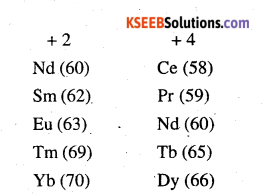• Ce after forming Ce4+ attains a stable configuration of [Xe]
• Tb after forming Tb4+ attains a stable configuration of [Xe] 4f7
• Eu after forming Eu2+ attains a stable configuration of [Xe] 4f7
• Yb after forming Yb2+ attains a stable configuration of [Xe] 4f14.

Question 33.
Compare the chemistry of the actinoids with that of lanthanoids with reference to :
(i) Electronic configuration
(ii) Oxidation states
(iii) Chemical reactivity.
(i) Electronic configuration: The actinoids involve the gradual filling of 5f-subshell in their atoms. Actinium has the outer electronic configuration of 6d17s2. From thorium (Z = 90) onward 5f-subshell gets progressively filled. Because of almost equal energies of 5fand 6d-subshells, there are some doubts regarding the filling of 5fand 6d-subshells.

(ii) Oxidation states Members of the actinoid family exhibit more variable oxidation states as compared to the elements belonging to lanthanoid family. This is due to the reason that there is less energy difference in 5d, 6fand 7s orbitals belonging to actinoid family than the energy difference in 5f, 4d and 65 orbitals in case of the lanthanoid family.

(iii) Chemical reactivity: Actinoids are highly reactive metals especially when these are in finely divided form. Upon boiling with water, they form respective oxides and hydroxides. They combine with a variety of non-metals at moderate temperature. On reacting with hydrochloric acid, they form their respective chlorides.Question 34.
Write the electronic configurations of the elements with the atomic numbers, 61, 91, 101, and 109.
61 : [Xe]54 4f5 5d0 6s2
91 : [Rn]86 5f2 6d1 7s2
101 : [Rn]86 5f13 5d0 7s2
109: [Rn]86 5f14 6d7 7s2 .

Question 35
Compare the general characteristics of the first series of transition metals with those of the second and third series metals in the respective vertical columns. Give special emphasis on the following points:
(i) electronic configurations
(ii) oxidation states
(iii) ionisation enthalpies and
(iv) atomic sizes.
(i) In the 1st, 2nd, and 3rd series of the transition metals the 3d, 4d, 5d orbitals are respectively filled.
We know that elements in the same vertical column generally have similar electronic configurations.
In the first transition series, two elements show unusual electronic configurations.

Cr (24): 3d5 4s1
Cu (29): 3d10 4s1

similarly, there are exception in 2nd transition series. These are

• Mo (42) : 4d5 5s1
• Tc (43) : 4d6 5s1
• Ru (44) : 4d7 5s1
• Rh (45) : 4d8 5s1
• Pd (46) : 4d10 5s1
• Ag (47) : 4d10 5s1.

There are exceptions in-3rd transition series also. These are

• W (74) : 5d4 6s2
• Pt (78) : 5d9 6s1
• Au (79) :5d10 6s1

As a result of these exceptions, it happens many times that the electronic configuration of the elements presents in the same group are dissimilar.

(ii) In each of the three transition series the number of oxidation states shown by the elements is the maximum in the middle and the minimum at the extreme ends. However, +2 and +3 oxidation states are quite stable for all elements present in the first transition series. All metals present in the first transition series form stable compounds in the +2 and +3 oxidation states. The stabilities of the +2 and +3 oxidation states decrease in the second and the third transition series, wherein higher oxidation states are more important.

For example, [FeII (CN)6]4- [COIII (NH3)6]3+, [Ti(H2O)6]3+ air-stable complexes, but no such complexes are known for the second and third transition series such as Mo, W, Rh, In. They form complexes in which their oxidation states are high. For example WCl6, ReF7, RuO4 etc.

(iii) In each of the three transition series, the first ionisation enthalpy increases from left to right. However, there are some exceptions. The first ionisation enthalpies of the third transition series are higher than those of the first and second transition series. This occurs due to the poor shielding effect of 4f electrons in the third transition series.

Certain elements in the second transition series have higher first ionisation enthalpies than elements corresponding to the same vertical column in the first transition series. There are also elements in the 2nd transition series whose first ionisation enthalpies are lower than those of the elements corresponding to the same vertical column in the 1st transition series.

(iv) Atomic sizes generally decrease from left to right across a period. Now, among the three transition series, the atomic sizes of the elements in the second transition series are greater than those of the elements corresponding to the same vertical column in the first transition series. However, the atomic sizes of the elements in the third transition series are virtually the same as those of the corresponding members in the second transition series this is due to lanthanoid contraction.

Question 36.
Write down the number of 3d electrons in each of the following ions:
Ti2+ , V2+ , Cr3+ , Mn2+ , Fe2+ , Fe3+ , CO2+ , Ni2+ and Cu2+ .
Indicate how would you expect the five 5d orbitals to be occupied for these hydrated ions (octahedral).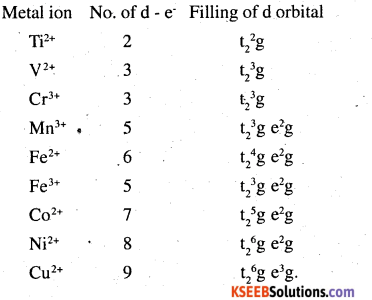Question 37.
Comment on the statement that elements of the first transition series possess many properties different from those, of heavier transition elements.
The above-mentioned statement is true, because
(a) Atomic radii of the heavier transition elements (4d and 5d series) are larger than .those of the corresponding elements of the first transition series though those of Ad and 5d series are very close to each other.
(b) Melting and boiling points of heavier transition elements are greater than those of the first transition series due to stronger intermetallic bonding.
(c) Enthalpies of atomisation of 4d and 5d series are higher than the corresponding elements of the first series.
(d) Ionization enthalpies of the 5d series are higher than the corresponding elements of the 3d and 4 d series.Question 38.
What can be inferred from the magnetic moment values of the following complex species?Magnetic moment (μ) is given asFor transition metals, the magnetic moment is calculated from the spin only formula Therefore
$$\sqrt{n(n+2)}$$ = 2.2

we can see from the above calculation that the given value is closest to n – 1. Also, in this complex,. Mn is in the +2 oxidation state. This means that Mn has 5 electrons in the d orbital. Hence, we can say that CN is a strong ligand that causes the pairing of electrons.

(ii) [Fe (H2O)6]2+
We. can see from the above calculation that µ= 5.3 is closest to the value when n = 4. Also, in this complex, Fe is in the +2 oxidation state. This means that Fe has 6 electrons in the d orbital. Hence, we can say that H2O is a weak field ligand and does not cause the pairing of e s.

(iii) K2 [Mncl4]
we can see that |X= 5.9 is closest to the value when n = 5. Also, in this computer, Mn is in the +2 oxidation state. This means that Mn has 5 electrons in the d-orbital. Hence we can say that Cl- is a weak ligand and does not cause the pairing of electrons.

### 2nd PUC Chemistry The d-and f-Block Elements Additional Questions and Answers

Question 1.
Some d-block elements are given below.
(Cr, Mn, Fe, Co, Ni)
(a) Identify the element which shows maximum paramagnetic behaviour.
(b) Give a reason for the highest paramagnetic property of that element.
(c) When two electrons are lost from each atom, is there any change in the magnetic properties of each? Explain?
(a) Cr
(b) Cr- 4s1 3d5
Mn – 4s2 3d5 .
Fe – 4s2 3d6
Co – 4s2 3d7
Ni – 4s2 3d8

Since there are six unpaired electrons in Cr, it has the maximum paramagnetic behaviour.
(c) Cr2+ →3d4
Mn2+ → 3d5
Fe2+ → 3d6
CO2+ → 3d7
Ni2+ → 3d8
when two electrons are lost, Mn2+ contains maximum number of unpaired electrons ie., Mn2+ is the ion with maximum paramagnetic behaviour. Cr2+ and Fe2+ show similar paramagnetism and so on.

Question 2.
On experimentation, it is found that, when salts of Cu+ ions are placed in a magnetic field, they are repelled by the magnetic field but that of Fe2+ ions are attracted by a magnetic field.

1. Briefly explain the chemistry behind it.
2. When the magnetic moment of a substance is determined, it shows a magnetic moment of 3.87 BM. How many unpaired electrons does it contain?
3. Most of the d-block elemental ions are paramagnetic. Why?

1. The salt of Cu+ ions is diamagnetic due to the absence of unpaired electrons while due to the presence of unpaired electrons in Fe2+, Fe2+ ions are paramagnetic and strongly attracted by the magnetic field.

2. Magnetic moment µ = $$\sqrt{n(n+2)}$$
But µ = 3.87 BM
ie., $$\sqrt{n(n+2)}$$ = 3.87
n(n + 2) = (3.87)2 = 15
This is possible when n = 3
ie., The substance contains 3 unpaired electrons.

3. Most of the d block elemental ions have unpaired electrons in the (n-1) d orbitals. Due to the presence of unpaired electrons in d orbitals, the transition metal ions become paramagnetic.

Question 3.
(a) What is blue Vitriol? How is it useful in detecting the presence of water vapour?
(b) When a magnetic field is applied, certain substances are attracted by the applied field. Those are called paramagnetic substances. Which is more paramagnetic Mn2+ or Cu2+? Why?
(a) CuSO4 5H2O is known as blue vitriol. Anhydrous copper sulphate is used for detecting the presence of water vapour. Water vapour converts white anhydrous copper sulphate to blue hydrated copper sulphate.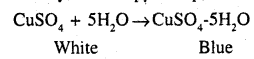(b) As the number of unpaired electrons increases, paramagnetism increases.
Mn → [Ar] 4s2 3d5 and Mn2+ → 3d5
Cu → [Ar] 4s1 3d10 and Cu2+ →3d9

Mn2+ contains five unpaired d-electrons and
Cu2+ contains an only unpaired electron.
∴ Mn2+ is more paramagnetic than 2+.Question 4.
(a) What do you observe when a dilute solution of KMnO4 is added in drops to a hot solution of dilute H2SO4? Is there any difference in observation when dilute KMnO4 is added to a mixture of hot dilute H2SO4 and oxalic acid?
(b) In the periodic table the atomic size increases down a group due to an increase in the number of shells. But 40Zn and 72HF in the 5th and 6th period respectively have almost identical radii explain?
(a) When dilute KMnO4 is added to a mixture of hot dilute H2SO4 and oxalic acid, brisk effervescence is formed due to the formation of CO2.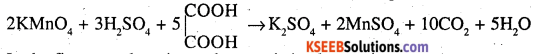In the first case, there is no characteristic observation

(b) The 4d and 5d series of transition elements in each group have the same atomic radii. This is due to lanthanide contraction.
The steady decrease in the size of the atoms or ions from lanthanum to lutetium is known as lanthanide contraction.

As the atomic number increases in the lanthanide series, the new electron goes to the inner 4f orbitals. The shielding effect of a 4f electron is poor. As a result, the attraction of the outer electrons by the nucleus increases and this tends to a decrease in size.

Question 5.
Mn exhibits a large number of oxidation states in its compounds. Which is the highest oxidation state?
Name an industrially important compound you have studied in which Mn is in its highest oxidation state. How is that compound manufactured?
In KMnO4, Mn exhibits the highest oxidation state of +7.
Oxygen and fluorine act as strong oxidising agents because of their high electronegativities and small sizes. Hence, they bring out the highest oxidation states from the transition metals. In other words, a transition metal exhibits oxidation states in oxides and fluorides. For example, in OsF6 and V2O5, the oxidation states of Os and V are + 6 and respectively.

Question 6.
(a) What may be the possible oxidation states of the transition metals with the following d electronic configurations for the ground state of their atoms 3d3 4s2, 3d5 4s2, and 3d6 4s2. Indicate the relative stability of oxidation states in each case.
(b) Write steps involved in the preparation of
(i) Na2CrO4 from chromite ore and
(ii) K2MnO4 from pyrolusite ore.
(a) The most stable oxidation state for 3d3 4s2 = +5
The most stable oxidation state for 3d5 4s2 = +7, +2
The most stable oxidation state for 3d6 4s2 = +2
In a transition series, the oxidation states which ‘ lead to exactly half-filled or completely filled d- orbitals are more stable. It shows that oxidation states +2 to +7 is most stable.

(b) i. The powdered chromite ore is heated with sodium carbonate in presence of air to get yellow coloured sodium chromite.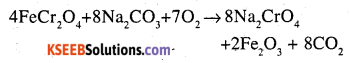ii. Finally powdered pyrolusite is fused with KOH in the presence of air to give green coloured potassium manganate

2MnO2 + 4 KOH +O2 → 2K2MnO4 + 2H2O.

Question 7.
Write complete chemical equations for
(i) Oxidation of Fe2+ by Cr2O72- in acid medium
(ii) Oxidation S2O32- by MnO4in a neutral aqueous medium
(i) Acidified potassium dichromate oxidises iron (II) salt to iron (III)
Cr2O72- + 14H + 6Fe2+ → 2Cr3+ + 6Fe3+ + 7H2O
(ii) In natural aqueous medium, thiosulphate
8MnO4+ 3s2O32- + H2O → 8MnO2+ 65O4 + 2OH

Question 8.
How would you account for the following situations
(i) The transition metals generally form coloured compounds.
(ii) With 3d4 configuration, Cr2+ acts as a reducing agent but Mn3+ acts as an oxidising agent (Atomic masses, Cr = 24, Mn = 25)
(iii) The actinoids exhibit a larger number of oxidation states than the corresponding lanthanoids.